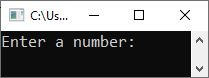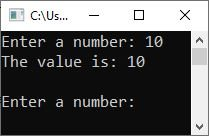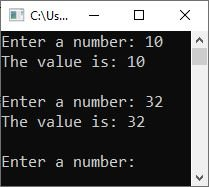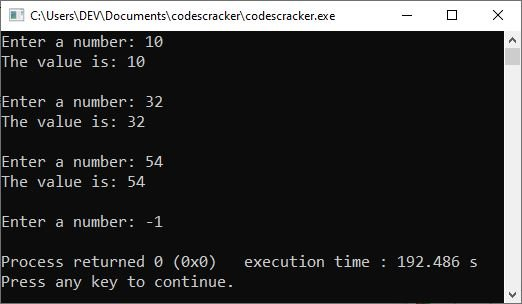# while loop in C programming with examples

This blog post was written and published to explain the "while" loop in the C programming language. So, without further ado, let's get started.

When we need to execute a block of code until the given condition evaluates to false, we use the "while" loop. The "while" loop takes the following general form:

```while(condition)
{
// body of the "while" loop
}```

That is, the "condition" will be evaluated first, and if it is true, the "body of the while loop" will be executed. The program flow evaluates the condition again after executing all of the statements available in the body of the "while" loop. If the condition is true again, the program flow enters the body of the loop and executes all of its statements. This procedure is repeated until the "condition" evaluates to false.

## while loop example in C

Consider the following program as an example of the "while" loop in C programming, which continues printing the value of "i" until the specified condition evaluates to false.

```#include <stdio.h>
int main()
{
int i = 1;
while(i<5)
{
printf("The value of 'i' = %d \n", i);
i++;
}

return 0;
}```

The output should be:

```The value of 'i' = 1
The value of 'i' = 2
The value of 'i' = 3
The value of 'i' = 4```

Unlike the "for" loop, the "while" loop's parameter only accepts one statement, which will be "condition_checking." The "initialization" must be done before the "while" loop, and the "update" statement must be written in the body of the "while" loop.

However, most of the time, C programmers use the "while" loop when they don't know the actual number of times a particular block of code needs to be executed. That is, when they wanted to execute a block of code until the specified condition evaluated to false, they preferred the "while" loop. Consider the following program:

```#include <stdio.h>
int main()
{
int num;

printf("Enter a number: ");
scanf("%d", &num);

while(num>0)
{
printf("The value is: %d", num);

printf("\n\nEnter a number: ");
scanf("%d", &num);
}

return 0;
}```

The following snapshot shows the initial output produced by the above program:Now type a number, say "10," and hit the ENTER key to see the following output:Type another number, say 32, and hit the ENTER key to see the following output:As you can see, the program continues to receive inputs from the user until the user enters a negative number or a zero. Consider the following snapshot for your understanding:And if you know the number of times to execute a block of code, use the "for" loop instead.

C Online Test

« Previous Tutorial Next Tutorial »